• +91 9971497814
• info@interviewmaterial.com

# Chapter 4 Linear Equations in Two Variables Ex-4.2 Interview Questions Answers

### Related Subjects

Question 1 :
Which one of the following options is true, and why?
y = 3x + 5 has
(i) a unique solution, (ii) only two solutions, (iii) infinitely many solutions

y = 3x + 5 is a linear equation in two variables and it has infinite possible solutions. As for every value of x, there will be a value of y satisfying the above equation and vice-versa.
Hence, the correct answer is (iii).

Question 2 :
Write four solutions for each of the following equations:
(i) 2x + y = 7 (ii) πx + y = 9 (iii) x = 4y

(i) 2x + y = 7

For x = 0,

2(0) + = 7

= 7

Therefore, (0, 7) is a solution of this equation.

For = 1,

2(1) + y = 7

y = 5

Therefore, (1, 5) is a solution of this equation.

For x = −1,

2(−1) + y = 7

y = 9

Therefore, (−1, 9) is a solution of this equation.

For = 2,

2(2) + y = 7

y = 3

Therefore, (2, 3) is a solution of this equation.

(ii) πx + y = 9

For x = 0,

π(0) + = 9

= 9

Therefore, (0, 9) is a solution of this equation.

For x = 1,

π(1) + = 9

= 9 −π

Therefore, (1, 9 − π) is a solution of this equation.

For x = 2,

π(2) + = 9

= 9 − 2π

Therefore, (2, 9 −2π) is a solution of this equation.

For x = −1,

π(−1) + = 9

= 9 + π

(−1, 9 + π) is a solution of this equation.

(iii) x = 4y

For x = 0,

0 = 4y

= 0

Therefore, (0, 0) is a solution of this equation.

For = 1,

x = 4(1) = 4

Therefore, (4, 1) is a solution of this equation.

For y = −1,

x = 4(−1)

x = −4

Therefore, (−4, −1) is a solution of this equation.

For x = 2,

2 = 4y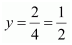Therefore,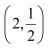is a solution of this equation.

Question 3 :

Check which of the following are solutions of the equation x −2= 4 and which are not:

(i) (0, 2 (ii) (2, 0) (iii) (4, 0)

(iv)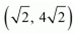(v) (1, 1)

(i) (0, 2)

Putting x = 0 and y = 2 in the L.H.Sof the given equation,

− 2y = 0 −2×2 = − 4 ≠ 4

L.H.S ≠ R.H.S

Therefore, (0, 2) is not a solution of this equation.

(ii) (2, 0)

Putting x = 2 and y = 0 in the L.H.Sof the given equation,

− 2y = 2 −2 × 0 = 2 ≠ 4

L.H.S ≠ R.H.S

Therefore, (2, 0) is not a solution of this equation.

(iii) (4, 0)

Putting x = 4 and y = 0 in the L.H.Sof the given equation,

− 2= 4 −2(0)

= 4 = R.H.S

Therefore, (4, 0) is a solution of this equation.

(iv)Putting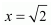and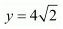in the L.H.S of the given equation,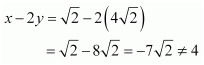L.H.S ≠ R.H.S

Therefore,is not a solution of this equation.

(v) (1, 1)

Putting x = 1 and y = 1 in the L.H.Sof the given equation,

− 2y = 1 −2(1) = 1 − 2 = − 1 ≠ 4

L.H.S ≠ R.H.S

Therefore, (1, 1) is not a solution of this equation.

Question 4 : Find the value of k, if x = 2, y = 1 is a solution of the equation 2x + 3y = k.

Putting x = 2 and y = 1 in the given equation,
2x + 3y = k
⇒ 2(2) + 3(1) = k
⇒ 4 + 3 = k
⇒ k = 7
Therefore, the value of k is 7.

Todays Deals### Chapter 4 Linear Equations in Two Variables Ex-4.2 Contributorskrishan

Name:
Email:

# Latest News# 9000 interview questions in different categories